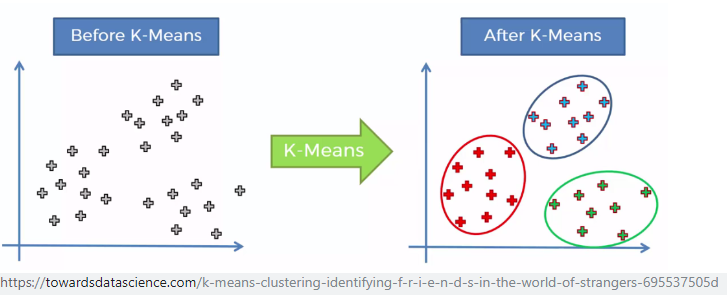# Accelerator for Machine Learning System

This project proposes building a designated accelerator, which efficiently performs RAM-to-RAM calculations in hardware in a pipeline fashion and thereby dramatically reducing CPU load for machine-learning software applications.An advanced scalable hardware accelerator for K-Means, targets unsupervised-learning and clustering applications.

Clustering is an unsupervised machine learning technique of dividing data into groups such that elements within each group are similar. Here every group is termed as cluster.

K-means clustering is one of the simplest and popular unsupervised machine learning algorithms.  However, finding the optimal solution to the k-means clustering problem for observations in d dimensions is NP-hard in general Euclidean space (of d dimensions) even just for 2 clusters:

∑ ∑ arg min || xj – ui ||2  (for all s where , i = 1..k , j ϵ Si )

where there are k clusters, Si is the cluster i, xj is the d-dimensional point in cluster Si and μi is the centroid (average of the points) of cluster Si.

There is a strong need for a hardware acceleration IP, to offload the CPU/MCU and allow a more power-efficient calculation approach.

This project proposes building a designated accelerator, which efficiently performs RAM-to-RAM calculations in hardware in a pipeline fashion and thereby dramatically reducing CPU load for machine-learning software applications. The accelerator is well defined in paper , provides APB interface  for external CPU access (configurations, results, etc.).

References